## Sunday, December 15, 2019

### Using `map.to.singleton` to plot a stochastic character map tree using `ape::plot.phylo`

It occurred to me today that it is both possible & relatively straightforward to plot a tree with a stochastic map character using ape's S3 `plot` method for the `"phylo"` object class by merely first converting the `"simmap"` object to a `"phylo"` object with singleton (that is, unbranching) nodes (which can already be done using `phytools::map.to.singleton`).

The main advantage of this is `ape::plot.phylo` has some options & plot styles that are not represented in `phytools::plot.simmap`.

Let's see.

First, here's our tree:

``````library(phytools)
tree
``````
``````##
## Phylogenetic tree with 26 tips and 25 internal nodes.
##
## Tip labels:
##  S, K, R, A, E, Z, ...
##
## The tree includes a mapped, 2-state discrete character with states:
##  0, 1
##
## Rooted; includes branch lengths.
``````
``````colors<-setNames(c("blue","red"),0:1)
plot(tree,colors,lwd=3)
``````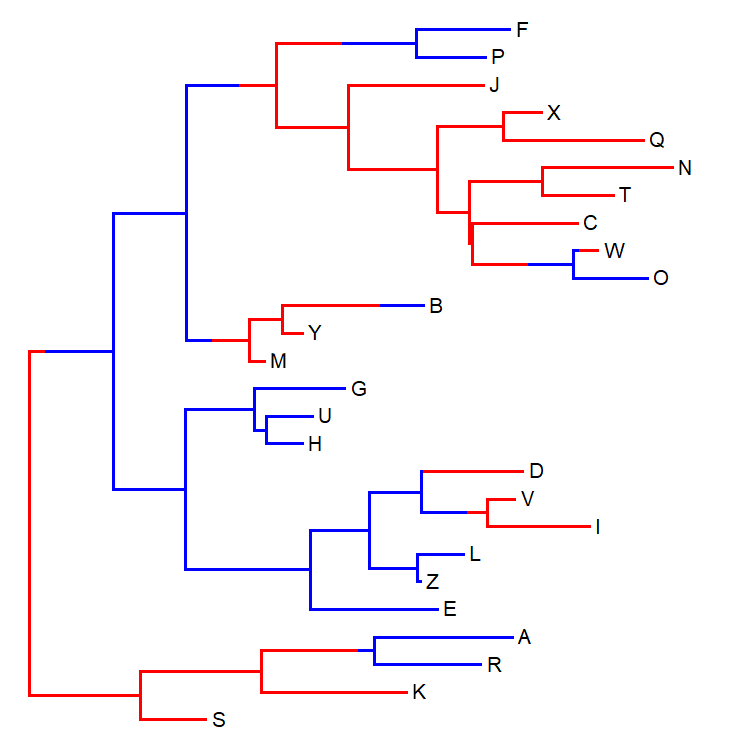Now, let's turn it in to a `"phylo"` object with unbranching nodes:

``````singles<-map.to.singleton(tree)
singles
``````
``````##
## Phylogenetic tree with 26 tips and 35 internal nodes.
##
## Tip labels:
##  S, K, R, A, E, Z, ...
##
## Rooted; includes branch lengths.
``````
``````plotTree.singletons(singles)
``````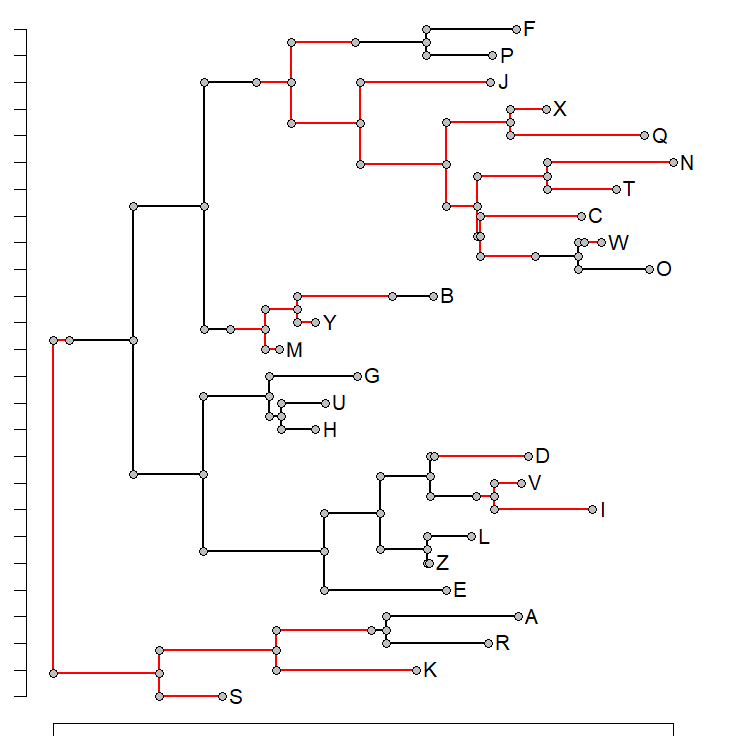Finally, let's replot it using `ape::plot.phylo`:

``````plot(singles,edge.col=colors[names(singles\$edge.length)],
edge.width=3,no.margin=TRUE)
``````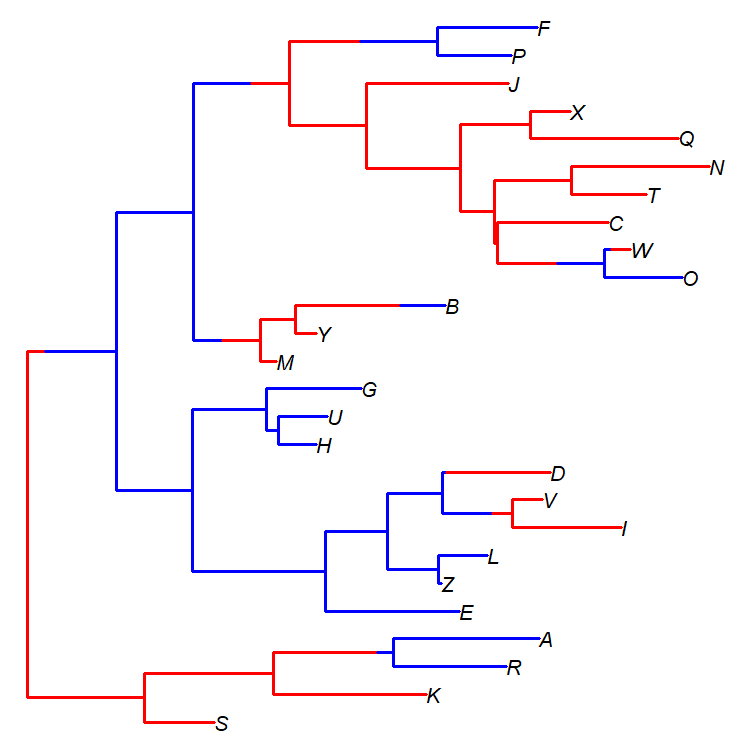Likewise, we can plot our tree in any of the different styles that `ape::plot.phylo` implements. For instance, an unrooted tree:

``````plot(singles,edge.col=colors[names(singles\$edge.length)],
edge.width=1,type="unrooted",lab4ut="axial",
cex=0.8,no.margin=TRUE)
``````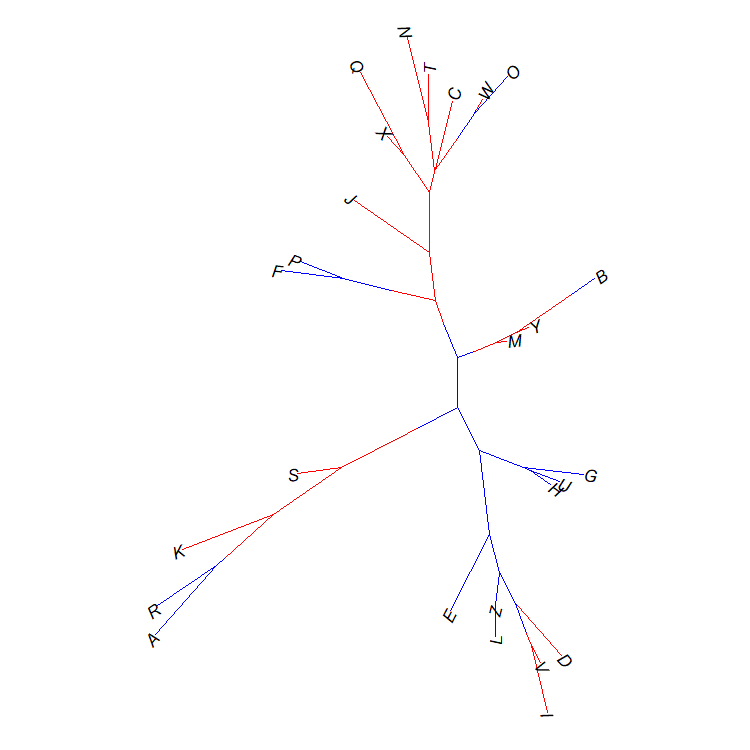Or, we can use other cool `plot.phylo` options, like plotting our different states with different line types instead of different colors:

``````par(lend=2)
types<-setNames(c("dotted","solid"),0:1)
greys<-setNames(c("black","grey"),0:1)
plot(singles,edge.lty=types[names(singles\$edge.length)],
edge.col=greys[names(singles\$edge.length)],
edge.width=4,no.margin=TRUE,label.offset=0.02)
``````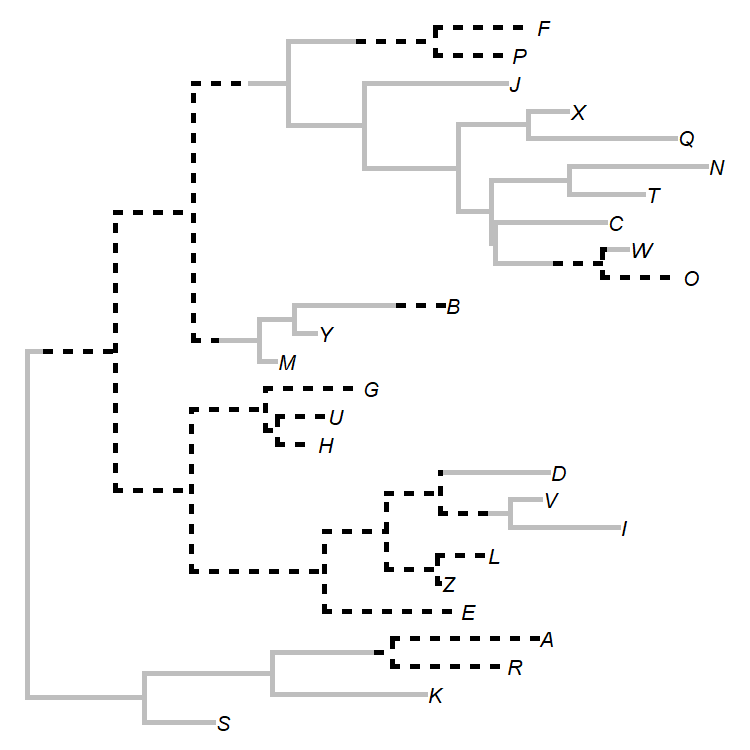That's kind of cool.

The tree & character history were simulated as follows:

``````set.seed(999)
Q<-0.5*matrix(c(-1,1,1,-1),2,2,dimnames=list(0:1,0:1))
tree<-sim.history(rtree(n=26,tip.label=LETTERS),Q)
``````